##### ENUM_RANGE() Function in PostgreSQL

PostgreSQL provides many built-in functions to manipulate the data having Enum data type. These functions include ENUM_FIRST(), ENUM_LAST(), and ENUM_RANGE(). Each of the functions serves a specific purpose, such as retrieving the first enumerator, last enumerator, etc.

This blog post will explain the working of the ENUM_RANGE() function in PostgreSQL.

## ENUM Functions in PostgreSQL

Postgres offers the following functions to deal with the enumerated data:

ENUM_FIRST() - This function returns the first enumerator of an enum type.

ENUM_LAST() - This function gives the last enumerator of an enum type.

ENUM_RANGE() - This function returns an array containing all the enumerators for the enum type in the same order as defined.

## What Does the ENUM_RANGE() Function Do in PostgreSQL?

ENUM_RANGE() is a built-in function in PostgreSQL that returns an array containing all the enumerators for the enum type in the same order as defined. The basic syntax for ENUM_RANGE() is given below:

enum_range(en_value ENUM);

Or if we write the detailed syntax for the function, it will be:

enum_range(en_range_start ENUM, en_range_end ENUM);

These parameters in the ENUM_RANGE() functions are mandatory to pass. Let's discuss the parameters one by one:

en_value - It is the enumeration value that is usually null.

en_range_start - It is the starting value of the enumeration range.

en_range_end - It is the ending/last value of the enumeration range.

Note: Here one thing is to notice that the en_range_start and en_range_end both should have the same enum type. And they can have a NULL value.

### What Does ENUM_RANGE() Function Return?

The ENUM_RANGE() function can return all the values or can also return a range of values in an enum.

If only one parameter is passed into the ENUM_RANGE() function, as written in the first syntax, it will give all the enumeration values in the particular enum type.

In the case of two parameters, these two parameters i.e. en_range_start and en_range_end will determine the range of the returned values. If the en_range_start is NULL then the range of the returning value will automatically be considered to start from the first enumeration value. If the en_range_end is NULL then the range of the returning value will automatically be considered till the end of the enumeration values. If both are NULL all the enumeration values will be returned.

Let’s see these cases one by one using an example so that they are more clear.

Example:

First of all, we need to create an enum type. Let’s consider it the name of the planets in the solar system. We will name the Enum as Solar_System:

```CREATE TYPE Solar_System AS ENUM (
'Mercury',
'Venus',
'Earth',
'Mars',
'Jupiter',
'Saturn',
'Uranus',
'Neptune',
'Pluto'
);```

By running the above query we will see that the Enum type has been created: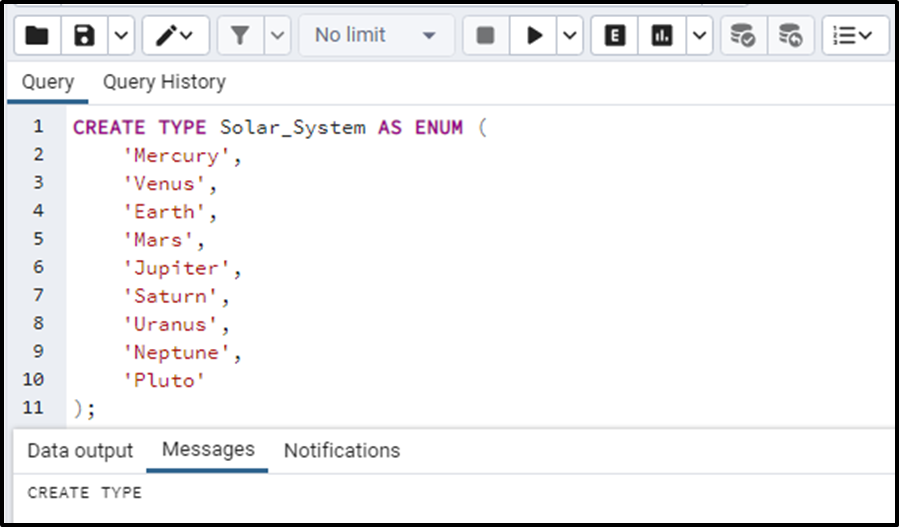Let's consider the cases for the different variations in the syntax of the enums and their values to evaluate the output.

### Case 1: One Parameter - NULL

Now let's consider the first case, the case of passing only one parameter in the function and that is set to be NULL. We will write the query as:

`SELECT enum_range(null::solar_system);`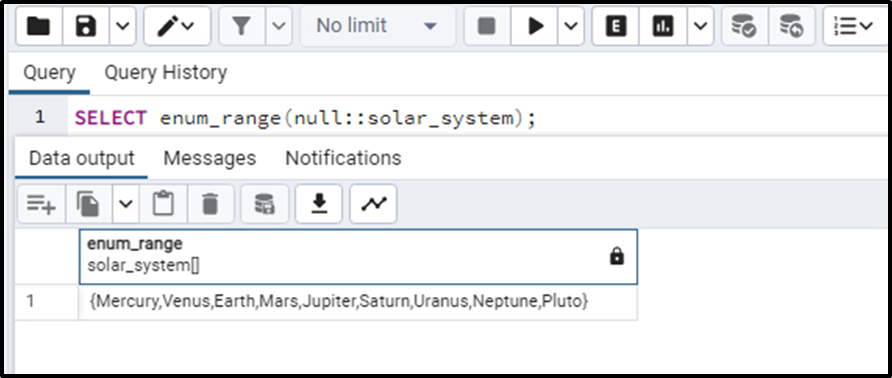We can see that passing NULL as one parameter outputs the whole enumeration values.

### Case 2: One Parameter - Non-null

Now if we pass a non-null value as a parameter.

```SELECT enum_range('Mercury'::solar_system),
enum_range('Venus'::solar_system),
enum_range('Earth'::solar_system),
enum_range('Mars'::solar_system),
enum_range('Jupiter'::solar_system),
enum_range('Saturn'::solar_system),
enum_range('Uranus'::solar_system),
enum_range('Neptune'::solar_system),
enum_range('Pluto'::solar_system);```

It will return all the enumerations.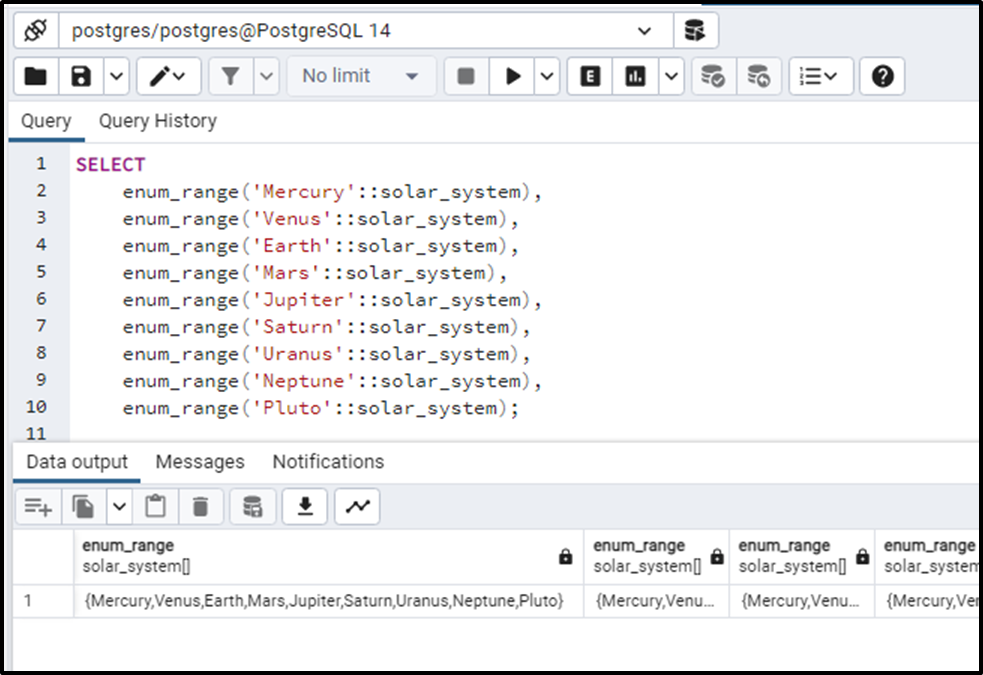Each query will result in all the enumeration values. So, the names of 9 planets will be returned 8 times. Now let's move to the two-parameter cases.

### Case 3: Two parameters - Both Parameters are Non-null

Let's consider the case where both the parameters i.e. en_range_start and en_range_end are non-null. It would give all the enum values between the range. Let's consider the following query:

`SELECT enum_range('Earth'::solar_system,'Uranus'::solar_system);`

The output for this query is as expected. It has returned all the enum values between the value “Earth” and “Uranus”.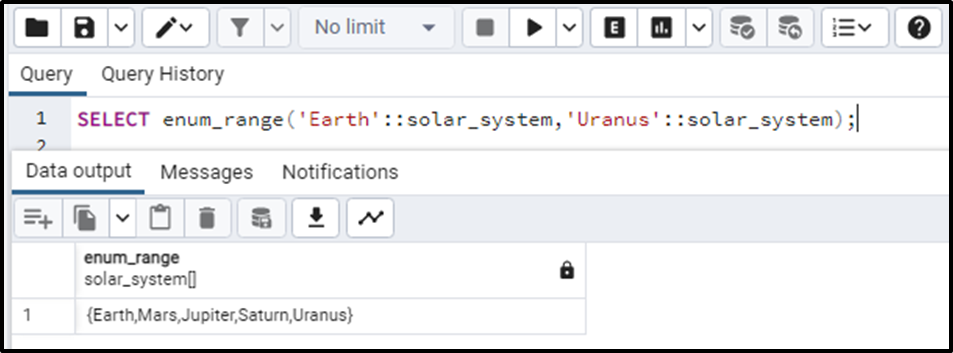### Case 4: Two Parameter - “en_range_start” is NULL

Now let's consider only the first parameter i.e. en_range_start is NULL. The query will be written as follows:

`SELECT enum_range(NULL::solar_system,'Saturn'::solar_system);`

The output for the above query will be the enum values starting from the start till the ”Saturn”. The output looks like this: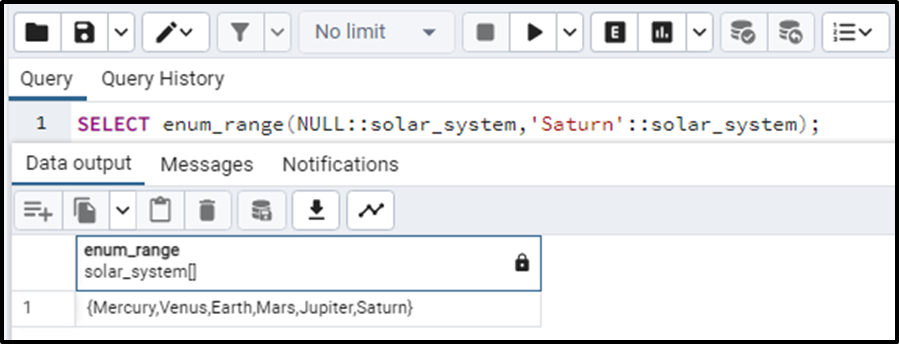### Case 5: Two Parameter - “en_range_end” is NULL

Now let's consider only the first parameter i.e. en_range_end is NULL. The query will be written as follows:

`SELECT enum_range('Venus'::solar_system, NULL::solar_system);`

The output for the above query will be the enum values starting from the ”Venus” till the end. The output looks like this: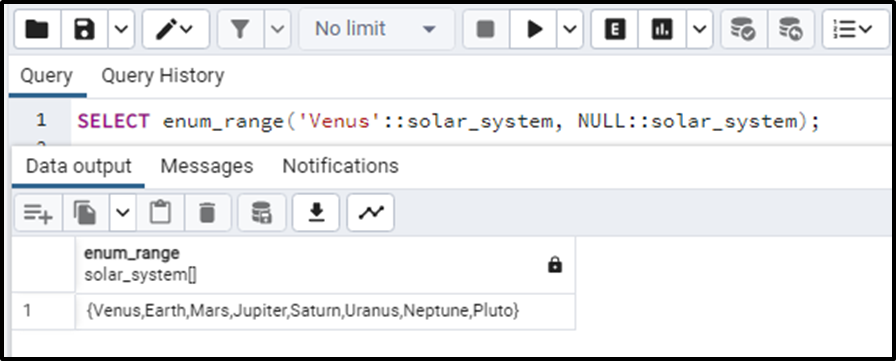### Case 6: Two parameters - Both Parameters are NULL

Now let’s see what happens if both the parameters are NULL. Execute the below query to find out:

`SELECT enum_range(NULL::solar_system, NULL::solar_system);`

We can clearly see that if both parameters are set to NULL, it will result in all the enumeration values: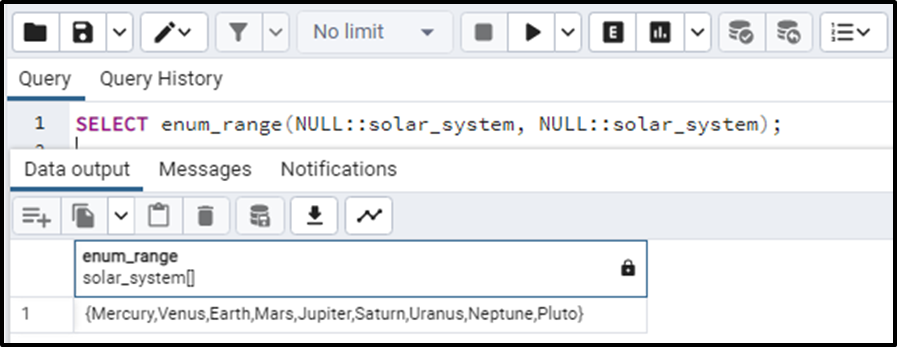So, this is all about the working of the ENUM_RANGE() in Postgres.

Conclusion

ENUM_RANGE() is a built-in function in Postgres to manipulate the data having Enum data type. This function returns an array containing all the enumerators for the enum type in the same order as defined. In this blog, we have learned about the details of the parameters and return values with examples.